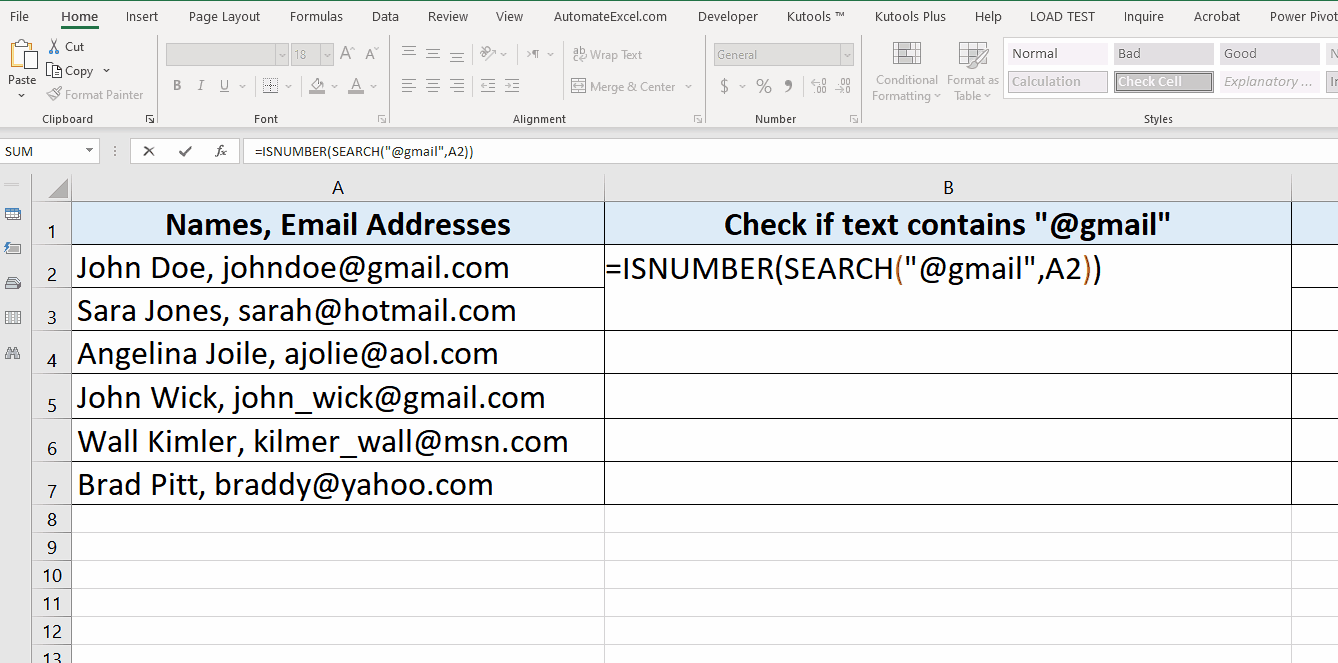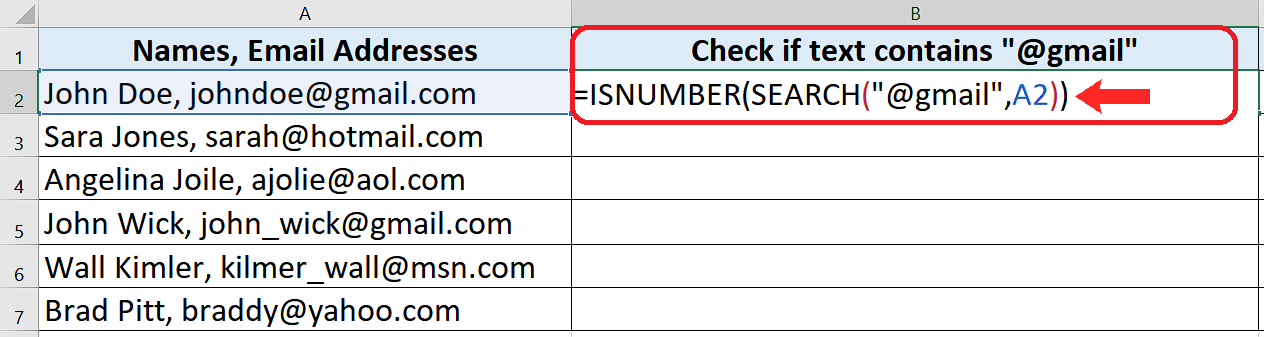# How to check if a string contains a specific word in Excel

Let’s take a look at a data set in the above picture which contains the names of people and email addresses within the same cell and we wish to find out which people have their email addresses on a particular domain.

Let’s dive into it to see how we can find a solution to our problem.

There is no direct formula to get to the solution, therefore we’ll use a combination of two formulas i.e. ISNUMBER() & SEARCH(). Let’s understand the logic first and then implement the formula.

SEARCH(substring, text)

Search function looks for a substring i.e. the part of a bigger text within the text. If the substring’s match is found then it returns a number, which is actually the starting position of that substring inside the complete text. If a match is not found then #Value error is returned.

So we use this to our advantage by using the results of the SEARCH function inside ISNUMBER() function. This function checks whether the input given to it is a number or not. If the input is a number then we get a TRUE result and FALSE otherwise.

So let’s implement this formula on our data set and see how to actually use both of these functions in the right combination.We often come across data which contains text and numbers together or only text in Excel. Sometimes it is necessary to know whether a text string contains a particular word or letter or not.

### Step 1 – Create the appropriate formula– The formula that we’ll use will be as follows;

=ISNUMBER(SEARCH(“@gmail”,A2)

### Step 2 – Implement the formula– The result of the formula will show that we got a TRUE result in every respective cell where the email address contained @gmail in it.

So this is how we can find out whether a given text contains a substring or not.